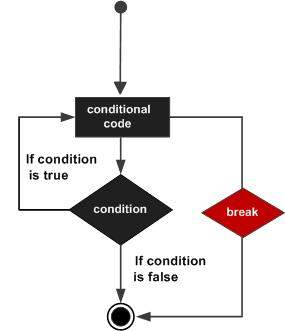R - Repeat Loop

The Repeat loop executes the same code again and again until a stop condition is met.

Syntax

The basic syntax for creating a repeat loop in R is −

repeat {
commands
if(condition) {
break
}
}

Flow DiagramExample

v <- c("Hello","loop")
cnt <- 2

repeat {
print(v)
cnt <- cnt+1

if(cnt > 5) {
break
}
}

When the above code is compiled and executed, it produces the following result −

 "Hello" "loop"
 "Hello" "loop"
 "Hello" "loop"
 "Hello" "loop"
r_loops.htm